# DAV Class 3 Maths Chapter 4 Worksheet 2 Solutions

The DAV Class 3 Maths Book Solutions and DAV Class 3 Maths Chapter 4 Worksheet 2 Solutions of Multiplication offer comprehensive answers to textbook questions.

## DAV Class 3 Maths Ch 4 WS 2 Solutions

Find the product:

Question 1.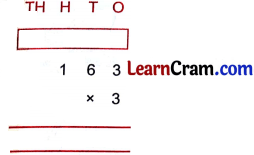Solution: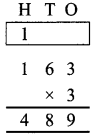Question 2.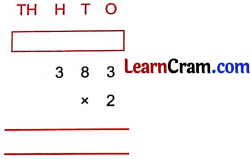Solution:Question 3.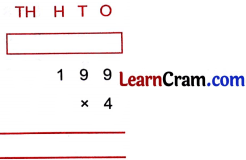Solution: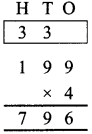Question 4.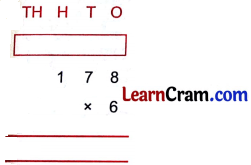Solution: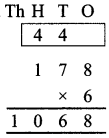Question 5.Solution:Question 6.Solution:Question 7.Solution:Question 8.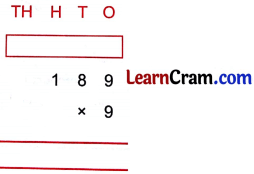Solution: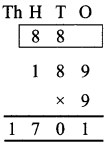Question 9.Solution: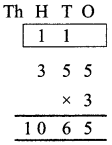Question 10.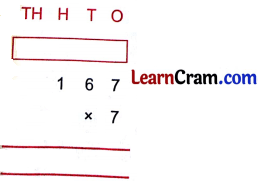Solution:Question 11.Solution: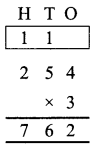Question 12.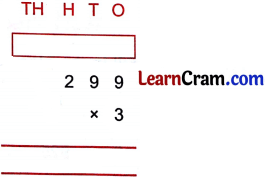Solution: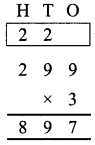### DAV Class 3 Maths Chapter 4 Worksheet 2 Notes

Step – 1:1. First multiply ones by 2.

Step – 2:2. Next multiply tens by 2 and carry 1 hundreds to hundreds place.Step – 3:3. Multiply hundred place number by 2. Add 1 hundred to it.